Question

A coil of 40 turns of wire is wound about a circular form. The average diameter of the individual loops is 30.5 cm. Calculate the magnetic moment of the coil when it carries a current of 2.50 A.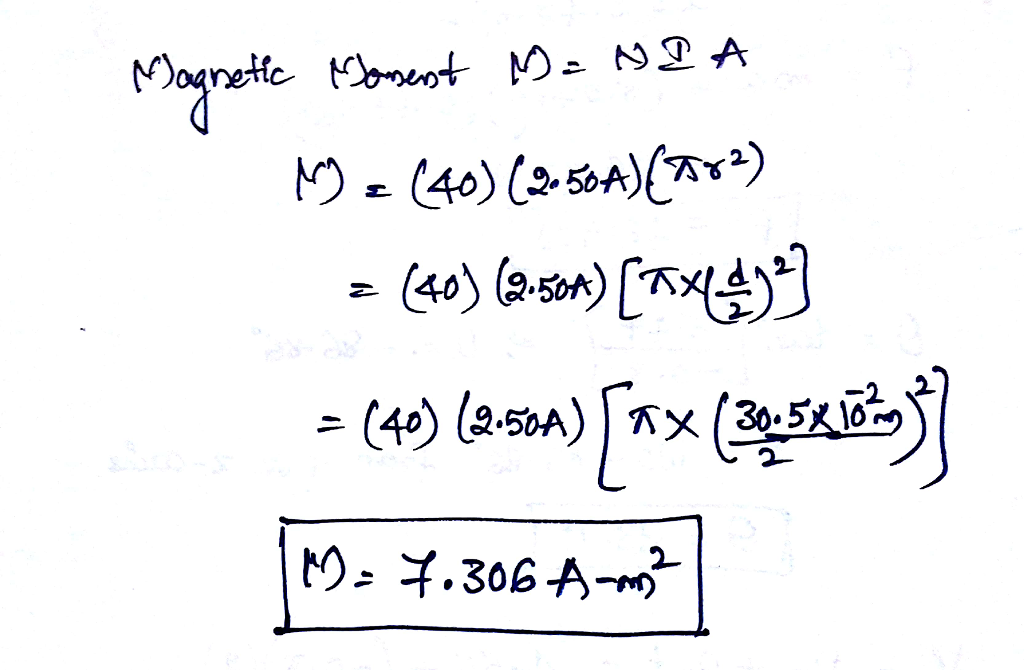#### Earn Coins

Coins can be redeemed for fabulous gifts.

Similar Homework Help Questions
• ### A closely wound circular coil with a diameter of 4.00 cm has 600 turns and carries...

A closely wound circular coil with a diameter of 4.00 cm has 600 turns and carries a current of 0.510 A . What is the magnetic field at the center of the coil?

• ### A closely wound circular coil with a diameter of 4.10 cm has 520 turns and carries...

A closely wound circular coil with a diameter of 4.10 cm has 520 turns and carries a current of 0.420 A. (a) What is the magnitude of the magnetic field at the center of the coil? T (b) What is the magnitude of the magnetic field at a point on the axis of the coil 8.00 cm from its center? T

• ### A closely wound circular coil with a diameter of 4.00cm has 600 turns and carries a current of...

A closely wound circular coil with a diameter of 4.00cm has 600 turns and carries a current of 0.500 A. What is the magnetic field at the center of the coil?

• ### Reviel A closely wound, circular coil with a diameter of 4.00 cm has 690 turns and...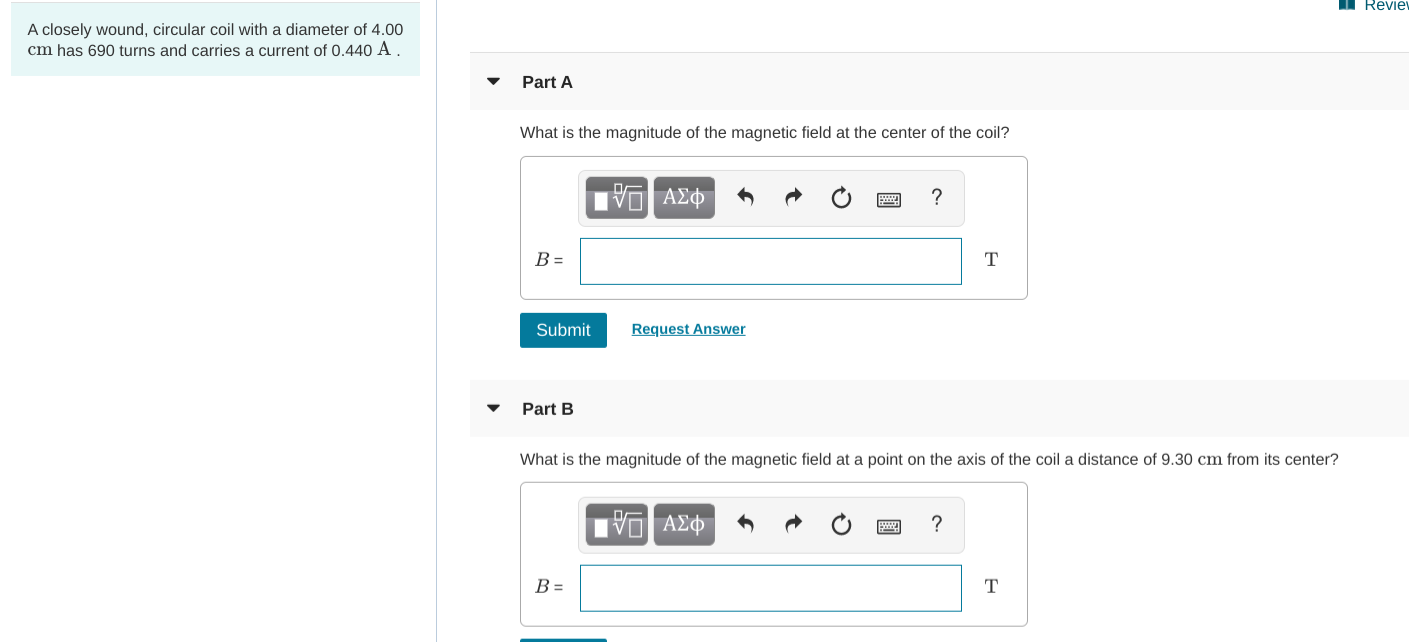Reviel A closely wound, circular coil with a diameter of 4.00 cm has 690 turns and carries a current of 0.440 A. Part A What is the magnitude of the magnetic field at the center of the coil? V AEO O ? B= Submit Request Answer Part B What is the magnitude of the magnetic field at a point on the axis of the coil a distance of 9.30 cm from its center? O AO O ?

• ### Thirty-seven turns of insulated wire 0.100 cm in diameter are tightly wound to form a flat...

Thirty-seven turns of insulated wire 0.100 cm in diameter are tightly wound to form a flat spiral. The spiral fills a disk surrounding a circle of radius 5.00 cm and extending to a radius 8.70 cm at the outer edge. Assume the wire carries a current I at the center of its cross section. Approximate each turn of wire as a circle. Then a loop of current exists at radius 5.05 cm, another at 5.15 cm, and so on. Numerically...

• ### A 75.0 Ω circular coil of wire of 25 turns, 15.00 cm in diameter, is rotated...

A 75.0 Ω circular coil of wire of 25 turns, 15.00 cm in diameter, is rotated at an angular velocity of 314 rad/s in an external magnetic field of 2.50 T. Find (a) the maximum induced emf, (b) the frequency of the alternating emf, (c) the maximum current in the coil, and (d) the instantaneous current at 7.00 s (in radians).

• ### A solenoidal coil with 30 turns of wire is wound tightly around another coil with 350...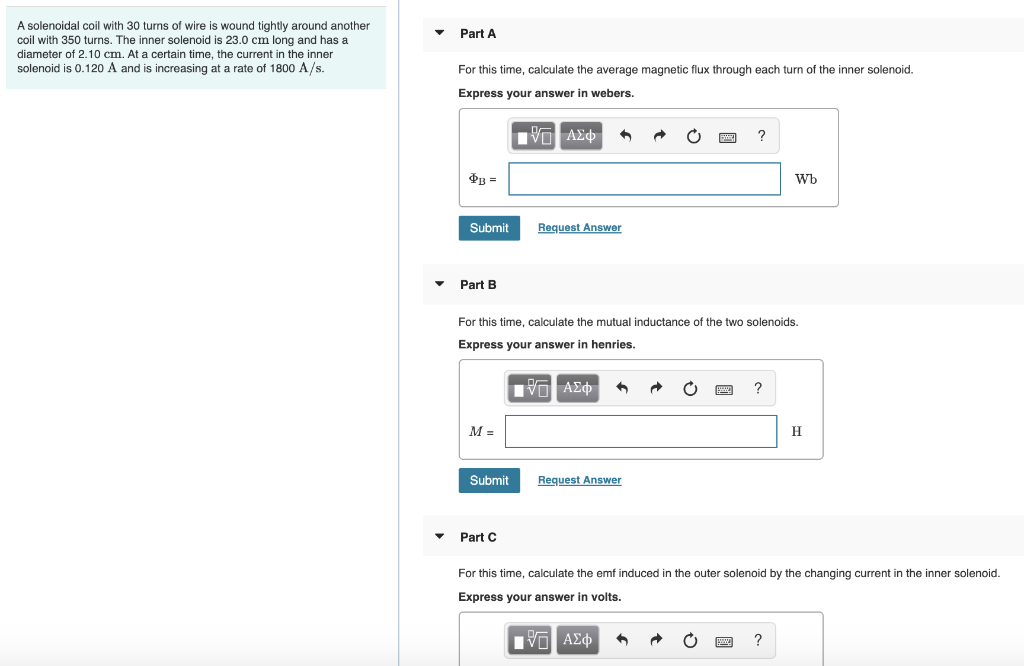A solenoidal coil with 30 turns of wire is wound tightly around another coil with 350 turns. The inner solenoid is 23.0 cm long and has a Part A diameter of 2.10 cm. At a certain time, the current in the inner solenoid is 0.120 A and is increasing at a rate of 1800 A/s. For this time, calculate the average magnetic flux through each turn of the inner solenoid. Express your answer in webers. Vη ΑΣφ' Фв - Wb...

• ### Part A A solenoidal coil with 25 turns of wire is wound tightly around another coil...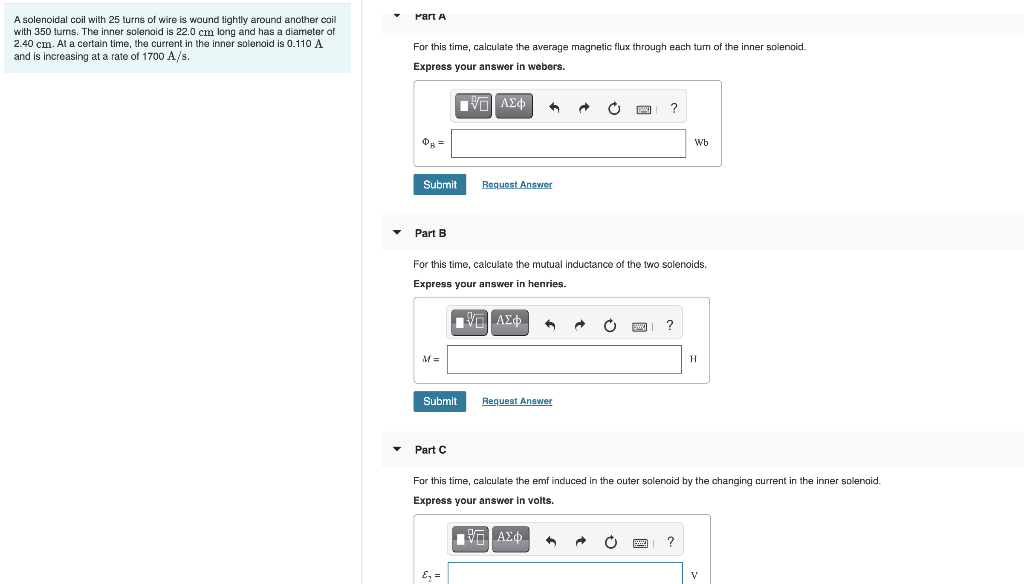Part A A solenoidal coil with 25 turns of wire is wound tightly around another coil with 350 turns. The inner solenoid is 22.0 cm long and has a diameter of 2.40 cm. At a certain time, the current in the inner solenoid is 0.110 A and is increasing at a rate of 1700 A/s. For this time, calculate the average magnetic flux through each turn of the inner solenoid. Express your answer in webers. Ivo A20 ? Wb Submit...

• ### A circular coil 14.0 cm in radius and composed of 145 tightly wound turns carries a...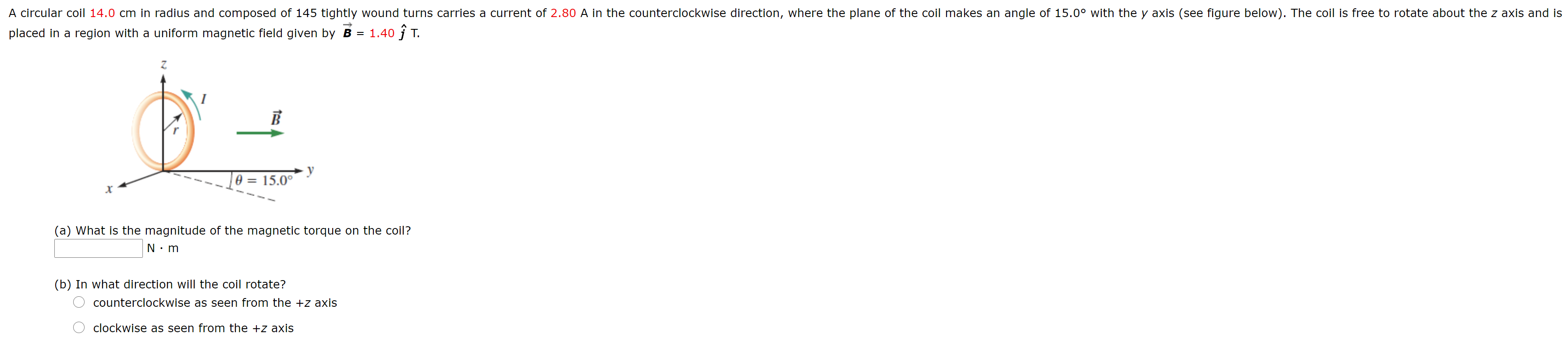A circular coil 14.0 cm in radius and composed of 145 tightly wound turns carries a current of 2.80 A in the counterclockwise direction, where the plane of the coil makes an angle of 15.0° with the y axis (see figure below). The coil is free to rotate about the z axis and is placed in a region with a uniform magnetic field given by B = 1.40 ſ T. B O = 15.0° (a) What is the magnitude of...

• ### A circular coil 13.0 cm in radius and composed of 145 tightly wound turns carries a...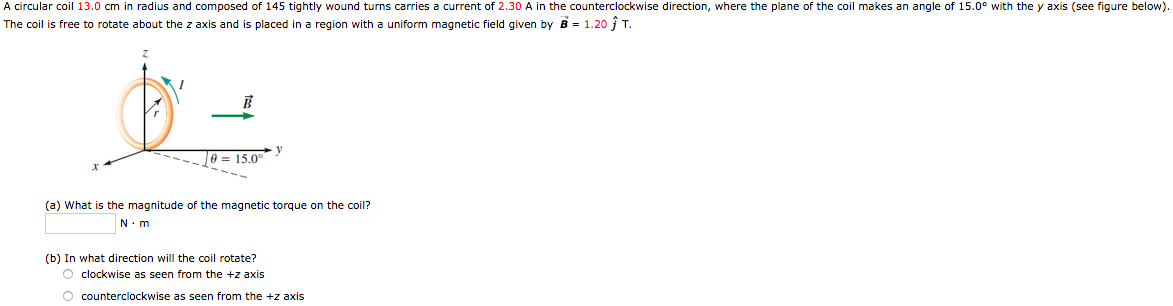A circular coil 13.0 cm in radius and composed of 145 tightly wound turns carries a current of 2.30 A in the counterclockwise direction, where the plane of the coil makes an angle of 15.0° with the y axis (see figure below). The coil is free to rotate about the z axis and is placed in a region with a uniform magnetic field given by B = 1.20 ſ T. Je = 15.0° (a) What is the magnitude of the...